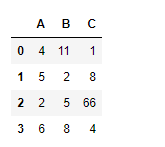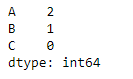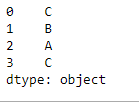Python | Pandas dataframe.idxmin()

• Last Updated : 19 Nov, 2018

Python is a great language for doing data analysis, primarily because of the fantastic ecosystem of data-centric python packages. Pandas is one of those packages and makes importing and analyzing data much easier.

Pandas dataframe.idxmin() function returns index of first occurrence of minimum over requested axis. While finding the index of the minimum value across any index, all NA/null values are excluded.

Syntax: DataFrame.idxmin(axis=0, skipna=True)

Parameters :
axis : 0 or ‘index’ for row-wise, 1 or ‘columns’ for column-wise
skipna : Exclude NA/null values. If an entire row/column is NA, the result will be NA

Returns : idxmin : Series

Example #1: Use idxmin() function to function to find the index of the minimum value along the index axis.

 # importing pandas as pdimport pandas as pd  # Creating the dataframe df = pd.DataFrame({"A":[4, 5, 2, 6],                   "B":[11, 2, 5, 8],                   "C":[1, 8, 66, 4]})  # Print the dataframedfNow apply the idxmin() function along the index axis.

 # applying idxmin() function.df.idxmin(axis = 0)

Output :If we look at the values in the dataframe, we can verify the result returned by the function. The function returned a pandas series object containing the index of minimum value in each column.

Example #2: Use idxmin() function to find the index of the minimum value along the column axis. The dataframe contains NA values.

 # importing pandas as pdimport pandas as pd  # Creating the dataframe df = pd.DataFrame({"A":[4, 5, 2, None],                    "B":[11, 2, None, 8],                    "C":[1, 8, 66, 4]})  # Skipna = True will skip all the Na values# find minimum along column axisdf.idxmin(axis = 1, skipna = True)

Output :My Personal Notes arrow_drop_up